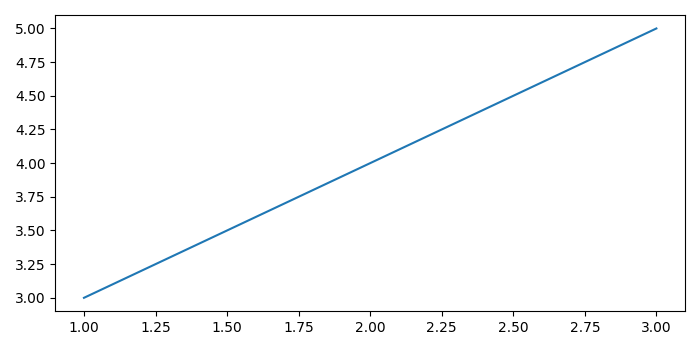# Correlation between two numeric columns in a Pandas DataFrame

We can use pandas.DataFrame.corr to compute pairwise correlation of columns, excluding NULL values. The correlation coefficient indicates the strength of the linear association between two variables. The coefficient ranges between -1 and 1.

To get the correlation between two numeric columns in a Pandas dataframe, we can take the following steps −

• Set the figure size and adjust the padding between and around the subplots.
• Create a Pandas dataframe of two-dimensional, size-mutable, potentially heterogeneous tabular data.
• Compare the values of the two columns and compute the correlation coefficient using col1.corr(col2).
• Print the correlation coefficient on the console.
• To display the figure, use show() method.

## Example

import pandas as pd
from matplotlib import pyplot as plt

plt.rcParams["figure.figsize"] = [7.00, 3.50]
plt.rcParams["figure.autolayout"] = True

df = pd.DataFrame({'lab': [1, 2, 3], 'value': [3, 4, 5]})

col1 = df['lab']
col2 = df['value']

plt.plot(col1, col2)

print("The correlation coefficient is: ", col1.corr(col2))

plt.show()

## Output

It will produce the following output

The correlation coefficient is: 1.0Here, the correlation coefficient is 1.0 which indicates perfect correlation. Hence, we get a straight line because all the points lie along a straight line.